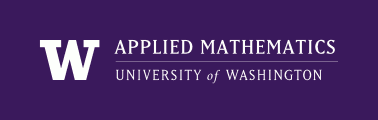AMath 584, Autumn Quarter, 2011 Applied Linear Algebra and Introductory Numerical Analysis

Some other references¶

Some of the books listed below are SIAM e-books and available free if you access them from a UW computer. Or if you are off-campus, you can gain access by using the UW library off-campus proxy.

See also October 13, 2011 Math library news for more tips on ebooks.

For more SIAM e-book titles related to numerical linear algebra, see Linear Algebra and Matrix Theory list (See also the list of SIAM textbooks on this topic).

Linear algebra¶

 [StrangBook] G. Strang, Introduction to Linear Algebra, Wellesley-Cambridge and SIAM, 2009.
 [StrangClass] G. Strang, Linear Algebra, free on-line course course from MIT. (Great instructor!)
 [Demmel] J. W. Demmel, Applied Numerical Linear Algebra, SIAM e-book, 1997.
 [Stewart-1] G. W. Stewart, Matrix Algorithms: Volume 1: Basic Decompositions, SIAM e-book, 1998.
 [Stewart-2] G. W. Stewart, Matrix Algorithms: Volume 2: Eigensystems, SIAM e-book, 2001.

Matlab¶

 [Driscoll] T. A. Driscoll, Learning MATLAB, SIAM e-book, 2009.
 [Higham] D. J. Higham and N. J. Higham, Matlab Guide: Second Edition SIAM e-book, 2005.
 [Moler] C. B. Moler, Numerical Computing with MATLAB, SIAM e-book, 2004.

Python¶

 [Dive-into-Python] M. Pilgram, Dive Into Python,.
 [Python] G. van Rossum, An Introduction to Python,

Latex¶

 [HighamChapter] N. J. Higham, Tex and Latex Chapter from: Handbook of Writing for the Mathematical Sciences, Second Edition, SIAM e-book. (See also Appendix B for latex symbols)
 [GriffithsHigham] D.F. Griffiths and D.J. Higham, Learning LaTeX, SIAM, 1997